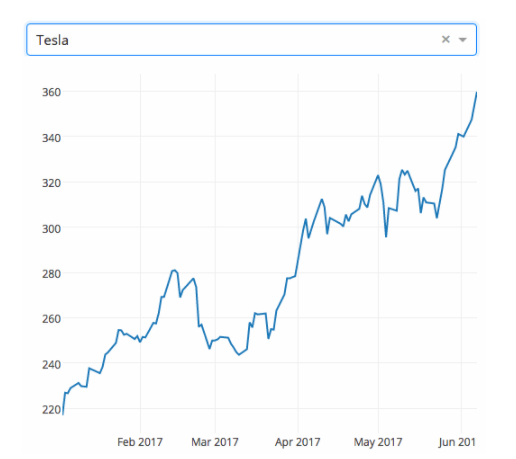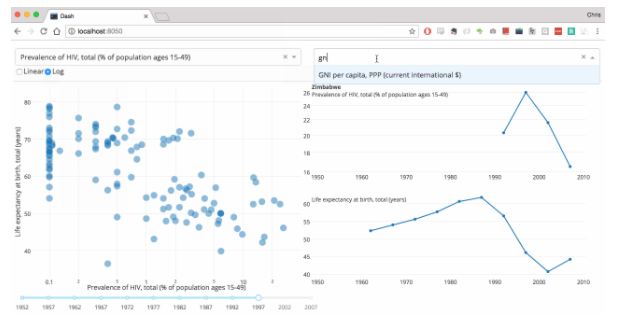• 写文章
• 提问

# Python数据可视化中dash怎么用？Dash

Dash```import dash
from dash.dependencies import Input, Output
import dash_core_components as dcc
import dash_html_components as html
from pandas_datareader import data as web
from datetime import datetime as dt
app = dash.Dash('Hello World')
app.layout = html.Div([
dcc.Dropdown(
id='my-dropdown',
options=[
{'label': 'Coke', 'value': 'COKE'},
{'label': 'Tesla', 'value': 'TSLA'},
{'label': 'Apple', 'value': 'AAPL'}
],
value='COKE'
),
dcc.Graph(id='my-graph')
], style={'width': '500'})
@app.callback(Output('my-graph', 'figure'), [Input('my-dropdown', 'value')])
def update_graph(selected_dropdown_value):
selected_dropdown_value,
dt(2017, 1, 1),
dt.now()
)
return {
'data': [{
'x': df.index,
'y': df.Close
}],
'layout': {'margin': {'l': 40, 'r': 0, 't': 20, 'b': 30}}
}
app.css.append_css({'external_url': 'https://codepen.io/chriddyp/pen/bWLwgP.css'})
if __name__ == '__main__':
app.run_server()```